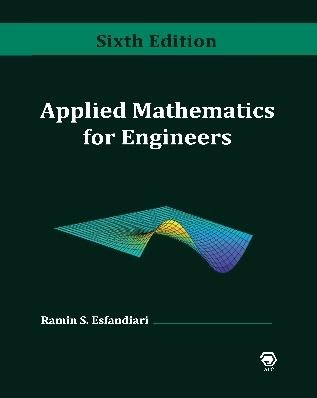## Atlantis Publishing Company (APC) Academic and Reference Textbooks

As a publisher of academic and reference textbooks, APC is committed to providing innovative and effective instructional materials for higher education.

#Applied Mathematics for Engineers, 6th Edition

## Book Description

• Price: U.S. \$149.99
• ISBN: 978-0-9729990-9-0
• 636 pp, hard cover, May 2021
• Inside this Book
Order

Applied Mathematics for Engineers, 6th edition, provides comprehensive knowledge of fundamental ideas and methods of applied mathematics utilized in various engineering disciplines. Each topic is covered in great detail, followed by meticulously worked-out examples, as well as a problem set containing a large number of additional related exercises. The book emphasizes on engineering applications to familiarize the reader with the physical laws and proper engineering terminology.

The book is divided into three major parts: The first part covers complex analysis, ordinary and partial differential equations, Laplace transformation, and Fourier analysis.

The second part presents a detailed coverage of matrix analysis and matrix eigenvalue problem.

The third part is devoted to numerical methods with applications in various engineering disciplines. MATLAB® is introduced and used to perform symbolic, graphical, and numerical tasks, ranging from basic curve fitting of data to numerical solution of initial-value problems and partial differential equations.

Topics

• Complex Analysis
• First-Order ODEs
• Second and Higher-Order ODEs
• Series Solution of ODEs
• Laplace Transformation
• Fourier Analysis
• Partial Differential Equations
• Matrix Analysis
• Matrix Eigenvalue Problem
• Introduction to MATLAB®
• Numerical Solution of Equations and Systems
• Curve Fitting and Interpolation
• Numerical Differentiation and Integration
• Numerical Solution of IVPs and BVPs
• Numerical Methods for the Eigenvalue Problem
• Numerical Solution of PDEs

Features

• Comprehensible pedagogy
• In-depth presentation of each topic
• Detailed solutions of examples and case studies
• Large set of exercises/projects for each topic
• MATLAB® user-defined functions for most methods